# A Set of 4 { Quad-Symmetric Based } Functions

## Summary of Findings

### Key Findings :

• We simply set-up 4 { Q-Functions } defined by :

•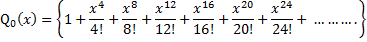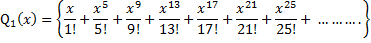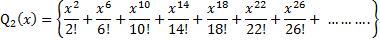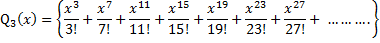so that :

•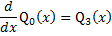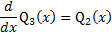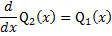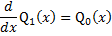And these 4 { Q-Functions } are { quad-symmetric based } to the extent that :

• the { derivative relations } are circular in nature , so that :

• any one of the 4 functions may be chosen the { lead-off } function for an analysis ,

and the result would be quite similar .

We can then express :

•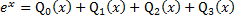and

•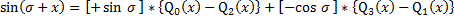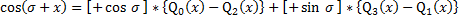If we take [ sigma ] as purely { real } and [ x ] as purely { imaginary } ,

• these 2 equations could possibly contribute to a finer understanding of the { sine } and { cosine } functions on the { complex plane } .

#### go to Top Of Page

 Link to Other Sections Section I The Search for { Quad-Symmetric Based } Functions Section II The 4 { Q-Functions } Section III Concluding Remarks